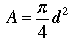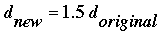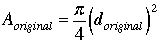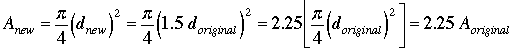SEARCH HOMEMath Central Quandaries & QueriesQuestion from mahwish, a student: A circular logo is enlarged to fit the lid of a jar. The new diameter is 50 per cent larger than the original. By what percentage has the area of the logo increased?Hello Mahwish,

Recall the formula for the area of a circle in terms of the diameter:The new diameter for the logo is 50% larger than the original, meaningThe area of the original logo isThe area of the new logo is,What does this mean in terms of percentages?

TylerMath Central is supported by the University of Regina and The Pacific Institute for the Mathematical Sciences.